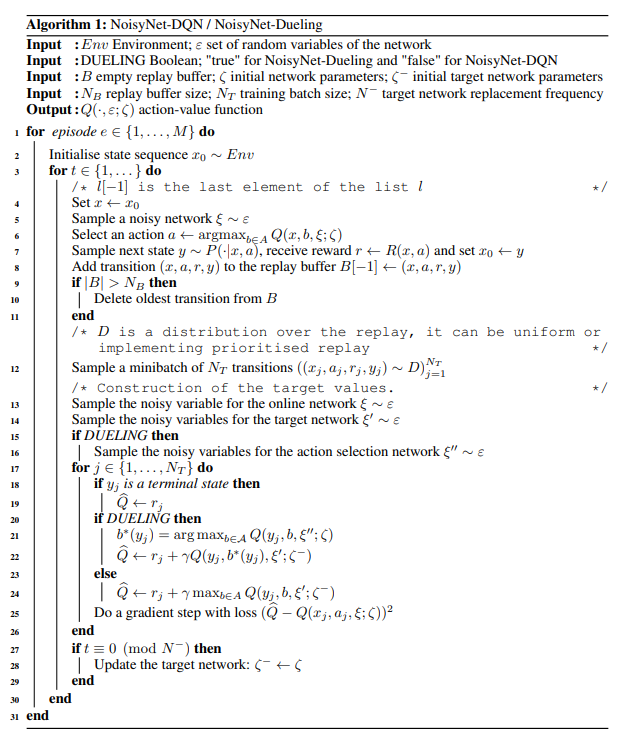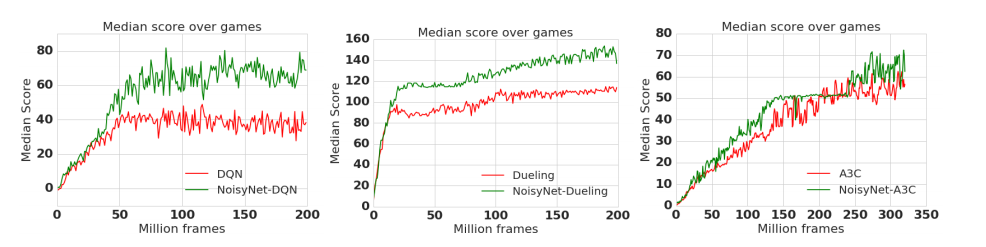# 强化学习的学习之路（二十六）_2021-01-26： Noisy DQN

y = w x + b y=w x+b

y = w x + b + ϵ y=w x+b+\epsilon

ϵ ∼ N ( 0 , σ 2 ) \epsilon \sim N\left(0, \sigma^{2}\right)
σ \sigma 是一个固定值，表示噪声带来的方差。这样我们也可以认为 y y 服火如下的高斯分布：
y ∼ N ( w x + b , σ 2 ) y \sim N\left(w x+b, \sigma^{2}\right)

w ~ ∼ N ( μ w , σ w ) b ~ ∼ N ( μ b , σ b ) y = w ~ x + b ~ \begin{array}{l} \tilde{w} \sim N\left(\mu_{w}, \sigma_{w}\right) \\ \tilde{b} \sim N\left(\mu_{b}, \sigma_{b}\right) \\ y=\tilde{w} x+\tilde{b} \end{array}

w ~ = μ w + σ w ϵ b ~ = μ b + σ b ϵ \begin{array}{l} \tilde{w}=\mu_{w}+\sigma_{w} \epsilon \\ \tilde{b}=\mu_{b}+\sigma_{b} \epsilon \end{array}

w ~ = μ w + σ w ϵ b ~ = μ b + σ b ϵ \begin{array}{l} \tilde{w}=\mu_{w}+\sigma_{w} \epsilon \\ \tilde{b}=\mu_{b}+\sigma_{b} \epsilon \end{array}

ϵ \epsilon 代表参数中的随机部分，它不属于参数, 服从确定的统计分布，例如均值为 0 , 0, 方差为 1 的高斯分布 N ( 0 , 1 ) N(0,1) 。在完成采样后，它可以被当成一个常量对待，这样另外两 个参数就可以使用前向后向计算优化了。当然，上面这种方法也只是为模型添加噪声的方法之一，如果利用这种方法增加噪声，那么如果函数原本有 N N 个参数，为了实现噪声的效果，我们需要把参数数量增加一倍，对于小型网络来说，使用这样的方法添加噪声是可行的，但对较大的网络来说，增加一倍的网络参数会给计算带来不小的负担。

w i , j = μ w [ i , j ] + f ( σ p [ i ] ) f ( σ q [ j ] ) ϵ \boldsymbol{w}_{i, j}=\mu_{w}[i, j]+f\left(\sigma_{p}[i]\right) f\left(\sigma_{q}[j]\right) \epsilon

b j = μ b [ j ] + f ( σ q [ j ] ) ϵ b_{j}=\mu_{b}[j]+f\left(\sigma_{q}[j]\right) \epsilon

L ( θ ) = E ( s t , a t , r t , s t + 1 ) ∼ D [ r t + γ max ⁡ a ∗ ∈ A Q ( s t + 1 , a ∗ ; θ − ) − Q ( s t , a t ; θ ) ] 2 L(\theta)=E_{\left(s_{t}, a_{t}, \boldsymbol{r}_{t}, \boldsymbol{s}_{t+1}\right) \sim D}\left[\boldsymbol{r}_{t}+\gamma \max _{\boldsymbol{a} * \in \boldsymbol{A}} Q\left(\boldsymbol{s}_{t+1}, \boldsymbol{a}^{*} ; \theta^{-}\right)-Q\left(s_{t}, \boldsymbol{a}_{t} ; \theta\right)\right]^{2}

L ( θ ) = E ϵ − , ϵ [ E ( s t , a t , r t , s t + 1 ) ∼ D [ r t + γ max ⁡ a ∗ ∈ A Q ( s t + 1 , a ∗ , ϵ − ; θ − , σ − ) − Q ( s t , a t , ϵ ; θ , σ ) ] 2 ] \begin{aligned} L(\theta)=& E_{\epsilon^{-}, \epsilon}\left[E_{\left(\boldsymbol{s}_{t}, \boldsymbol{a}_{t}, \boldsymbol{r}_{t}, \boldsymbol{s}_{t+1}\right) \sim D}\left[\boldsymbol{r}_{t}+\gamma \max _{\boldsymbol{a} * \in \boldsymbol{A}} Q\left(\boldsymbol{s}_{t+1}, \boldsymbol{a}^{*}, \epsilon^{-} ; \theta^{-}, \sigma^{-}\right)\right.\right.\\ &\left.\left.-Q\left(\boldsymbol{s}_{t}, \boldsymbol{a}_{t}, \epsilon ; \theta, \sigma\right)\right]^{2}\right] \end{aligned}02-2562
02-262804-261868
07-151万+
02-2641
02-2615
02-2641
01-06232
02-2675
01-1573
02-2651
02-2533
02-2620
09-02
07-26375
07-13378
01-2361
12-06509
11-3022
©️2020 CSDN 皮肤主题: 大白 设计师:CSDN官方博客